# B. Driving Force Discretization

TO IMPLEMENT the discretization scheme described in Chapter 3 into the device simulator MINIMOS-NT an expression for the driving force was required. The driving force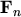is defined by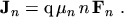(B.1)

To obtain the discrete driving force the discretized current density eqns. (3.63) and (3.64)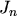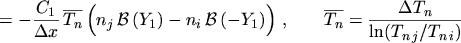(B.2)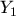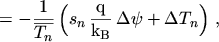(B.3)

must therefore be divided in some way by the electron concentration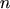. Thus, an average carrier concentration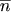is introduced via the following definition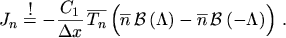(B.4)

By comparing the coefficients of eqn. (B.4) with those from eqn. (B.2)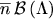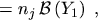(B.5)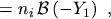(B.6)

and using the identity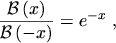(B.7)

the new argument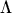of the BERNOULLI function can be calculated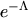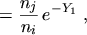(B.8)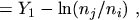(B.9)

and the average carrier concentration is finally found to be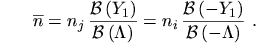(B.10)

Applying the identity(B.11)

to eqn. (B.4) yields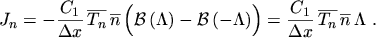(B.12)

After inserting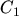from eqn. (2.188)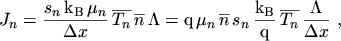(B.13)

the expression for the discretized driving force can easily be obtained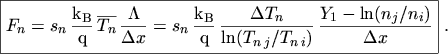(B.14)

The consistency of the discretization can be checked by calculating the driving force in the limit of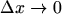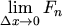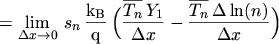(B.15)(B.16)

where the abbreviations forandhave been expanded. Using the total derivative yields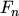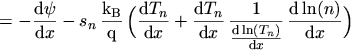(B.17)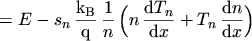(B.18)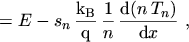(B.19)

which is the one-dimensional projection of the driving force(B.20)

M. Gritsch: Numerical Modeling of Silicon-on-Insulator MOSFETs PDF